﻿ Single Digit Subtraction Fluency Worksheets

Single Digit Subtraction Fluency Worksheets

Single Digit Subtraction Fluency Worksheets, Subtraction Facts Within TwentySubtraction Numberline 2

Common Core State Standards: CCSS 2.OA.2 Fluently add and subtract within 20 using mental strategies,...
Numberline with arrows for subtraction.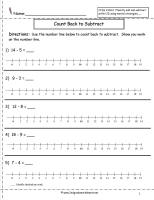Numberline Subtraction Worksheet2, 3,4,

Common Core State Standards: CCSS 2.OA.2 Fluently add and subtract within 20 using mental strategies,...
Five subtraction problems with dedicated numberline to teach subtraction.Numberline Subtraction Worksheet 2,3,4, 5

Common Core State Standards: CCSS 2.OA.2 Fluently add and subtract within 20 using mental strategies,...
18 subtraction problems. Numberline included.Numberline Subtraction 2, 3, 4, 5

Common Core State Standards: CCSS 2.OA.2 Fluently add and subtract within 20 using mental strategies,...
20 verticalsubtraction problems. Numberline included.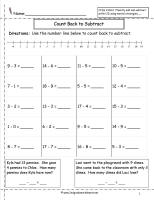Numberline Subtraction with Word Problems Worksheet

Common Core State Standards: CCSS 2.OA.2 Fluently add and subtract within 20 using mental strategies,...
25  horizontal subtraction problems. Word problems included.  Numberline included.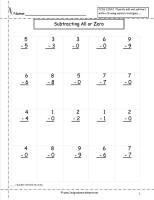Subtracting All or Zero Worksheet

Common Core State Standards: CCSS 2.OA.2 Fluently add and subtract within 20 using mental strategies,...
20 subtraction problems.Subtracting One Worksheet

Common Core State Standards: CCSS 2.OA.2 Fluently add and subtract within 20 using mental strategies,...
25 subtraction problems.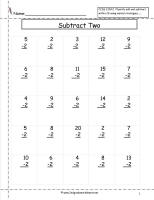Subtracting Two Worksheet

Common Core State Standards: CCSS 2.OA.2 Fluently add and subtract within 20 using mental strategies,...
25 subtraction problems.Subtracting Three Worksheet

Common Core State Standards: CCSS 2.OA.2 Fluently add and subtract within 20 using mental strategies,...
25 subtraction problems.Subtracting Four Worksheet

Common Core State Standards: CCSS 2.OA.2 Fluently add and subtract within 20 using mental strategies,...
25 subtraction problems.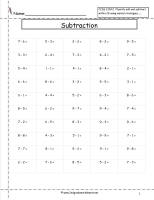Mixed Subtraction Facts Worksheet

Common Core State Standards: CCSS 2.OA.2 Fluently add and subtract within 20 using mental strategies,...
50  horizontal subtraction problems.Mixed Subtraction Review

Common Core State Standards: CCSS 2.OA.2 Fluently add and subtract within 20 using mental strategies,...
Mixed subtraction review worksheet.